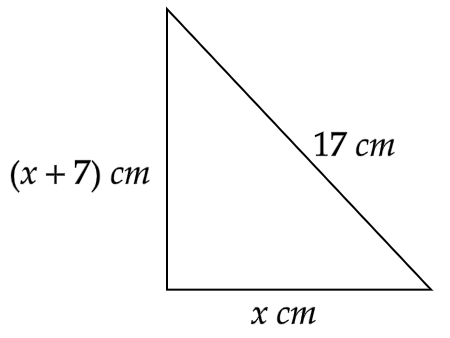# The hypotenuse of a right triangle is $17{\text{ }}cm$ long. Another side of the triangle is $7{\text{ }}cm$ longer than the third side. How do you find the unknown side lengths?Verified
94.5k+ views
Hint: Given, the hypotenuse of a right triangle is $17{\text{ }}cm$ long and another side of the triangle is $7{\text{ }}cm$ longer than the third side. So, we will assume the other two sides to be $x{\text{ }}cm$ and $\left( {x + 7} \right){\text{ }}cm$. Then we will apply Pythagoras theorem in the given triangle. We will obtain a quadratic equation in $x$. On solving the quadratic equation, we will find the unknown side lengths.

Given, the length of the hypotenuse is $17{\text{ }}cm$. Also, given that another side of the triangle is $7{\text{ }}cm$ longer than the third side. So, we will assume the other two sides to be $x{\text{ }}cm$ and $\left( {x + 7} \right){\text{ }}cm$.Pythagoras theorem states that the square of the hypotenuse is equal to the sum of the square of the two legs of the right-angle triangle.
Using the Pythagoras theorem, we get
$\Rightarrow {\left( {17} \right)^2} = {\left( x \right)^2} + {\left( {x + 7} \right)^2}{\text{ }}$
On squaring, we get
$\Rightarrow 289 = {x^2} + {x^2} + 14x + 49$
$\Rightarrow 289 = 2{x^2} + 14x + 49$
Subtracting $49$ from both the sides and on rewriting, we get
$\Rightarrow 2{x^2} + 14x = 240$
$\Rightarrow 2{x^2} + 14x - 240 = 0$
On dividing both the sides by $2$, we get
$\Rightarrow {x^2} + 7x - 120 = 0$
On splitting the middle term, we get
$\Rightarrow {x^2} + 15x - 8x - 120 = 0$
On taking common, we get
$\Rightarrow x\left( {x + 15} \right) - 8\left( {x + 15} \right) = 0$
$\Rightarrow \left( {x - 8} \right)\left( {x + 15} \right) = 0$
So, we get,
$\Rightarrow x = 8$ or $x = - 15$
But as we know that length can’t be negative. So, $x \ne - 15$.
Therefore, we get $x = 8$ and $x + 7 = 15$.
Therefore, the lengths of unknown sides are $8{\text{ }}cm$ and ${\text{15 }}cm$.

Note: The two perpendicular sides of a right triangle are called the legs. In an isosceles right triangle, the two legs are of equal length. So, the corresponding angles are also congruent. The corresponding angles are $45$ degrees each. For any right-angle triangle, Pythagoras theorem is the most important formula.